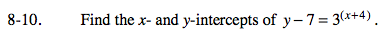### Home > A2C > Chapter 8 > Lesson 8.1.1 > Problem8-10

8-10.

Find the x- and y-intercepts of y − 7 = 3(x+4). Homework Help ✎Set x to 0 and solve to find the y-intercept, and set y to 0 and solve to find the x-intercept.

No x-intercept
y-intercept: (0, 88)